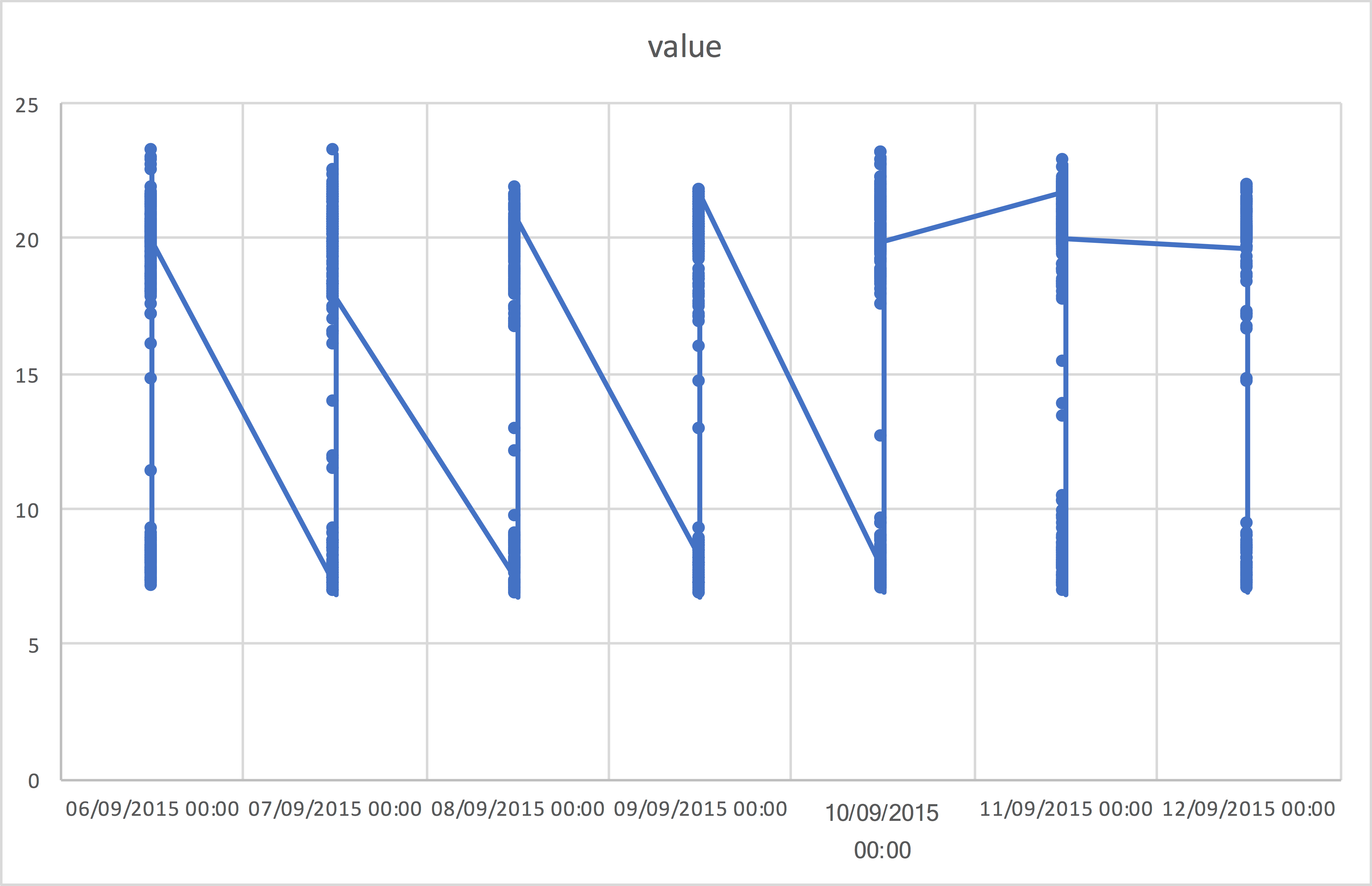# Calculator < Spreadsheet < R < ? for large n

It has previously been suggested that the introduction double entry accounting funded the renaissance. This seems quite illogical at first sight. How can a change to an accounting method produce more money? I suspect that the answer is that the new method reduced error and thus reduced the number of petty disputes. It also made theft harder for employees. With the new system, less checking was needed; trust was increased; supplier and consumer were in greater harmony. The point is: a new method, even for something as simple as adding numbers, can have a remarkable effect.

I recently watched my sister use a calculator on an Ipad to add up the numbers on a bill to check them. Initially, I was impressed by her fiscal diligence. Being me, I tried to convince her to use the spreadsheet that she has built in to her Ipad. She was quite unimpressed with this idea and kept using her Ipad calculator.

And then the numbers didn’t add up. Fortunately, I wasn’t so silly as to keep pressing her to use a spreadsheet and also fortunately, the calculator app being used had a history. It showed that a sneaky “zero times” had been inserted at the front.

The lesson is that, for small tasks, calculators (and mental arithmetic) are just fine but they don’t scale. As soon as the size of the problem increases (even slightly) one is soon left with repeating the calculation several times to check that you have the right answer.

It’s interesting to see that spreadsheet tools have a similar problem.

During a writing course that I teach to engineering and computer science PhD students, I ask them to take some real-life data, involving temperatures in different rooms of a house over time, and produce a meaningful graph. Since the data have more than two dimensions (room, time, temperature) producing a graph with most spreadsheet tools is difficult. Interestingly, very few of the otherwise well-educated students manage to produce a correct graph. Most often, they get a graph like this:This particular graph is wrong because it doesn’t distinguish between different rooms and truncates the date value incorrectly. Spreadsheet tools produce a wrong graph easily. Unfortunately, it is almost impossible to get such tools to produce a correct graph.

R, and in particular the ggplot2 library for producing graphs, are much better tools for this task. Here is one solution as R code.

```library(ggplot2)
library(dplyr)
x <- read_csv("getData.csv", skip = 2,
col_names=c("nodeId", "house","room","time","value"))
y <- mutate(x, time= as.POSIXct(time, format="%d/%m/%Y %H:%M:%S", tz="GMT"))
ggplot(y, aes(x=time, y=value, colour=room)) +
geom_point() + geom_line()```

This code looks complicated but it’s been created step by step in Rstudio, a tool that makes it easy to construct the code and test each piece as you go.

Here are the key steps:

1. read the data into memory
2. convert / massage data
3. plot the massaged data

Step one is to read in the data into memory. I prefer `read_csv` from the readr package because it’s fast and it doesn’t make too many assumptions or try to interpret the data. A first attempt might look like:

```library(readr)

This almost works but the extra header lines cause a problem. `skip = 2` gets rid of these but loses the header information so a simple fix is to manually specify the column names with `col_names`.

The next step is to convert the time from a string to a time / date format. R has a number of ways of storing times but POSIXct, which stores date and time as seconds since 1st Jan 1970, is usually a good choice. Note that when converting, you need to say what timezone is involved. Since the data was gathered in the UK, GMT is probably a safe bet.

The conversion requires a format string. This is another improvement over the spreadsheet tools, which guess the format and sometimes wrongly choose M/D/Y when the date is really D/M/Y.

With R, we have a variety of ways to update a column. The following might look simpler to some:

`x\$time <- as.POSIXct(x\$time, format="%d/%m/%Y %H:%M:%S", tz="GMT")`

This uses `\$` to reference a data frame column by name.

This syntax is a bit unreadable though and dplyr offers a nice alternative. For this short script, either will do.

The final step is to plot the data. R has in-built plotting tools but ggplot2 provides much more flexibility. We start by telling it which data frame and what axes. Note that the axes parameters go inside `aes()` (short for aesthetic).

`ggplot(y, aes(x=time, y=value, colour=room)) + geom_point() + geom_line()`

ggplot() on its own doesn’t produce a plot. For that, we need to add a layer. Here, we’ve added two: one that produces a point for each data point and one that draws lines between the points. The result looks like this: